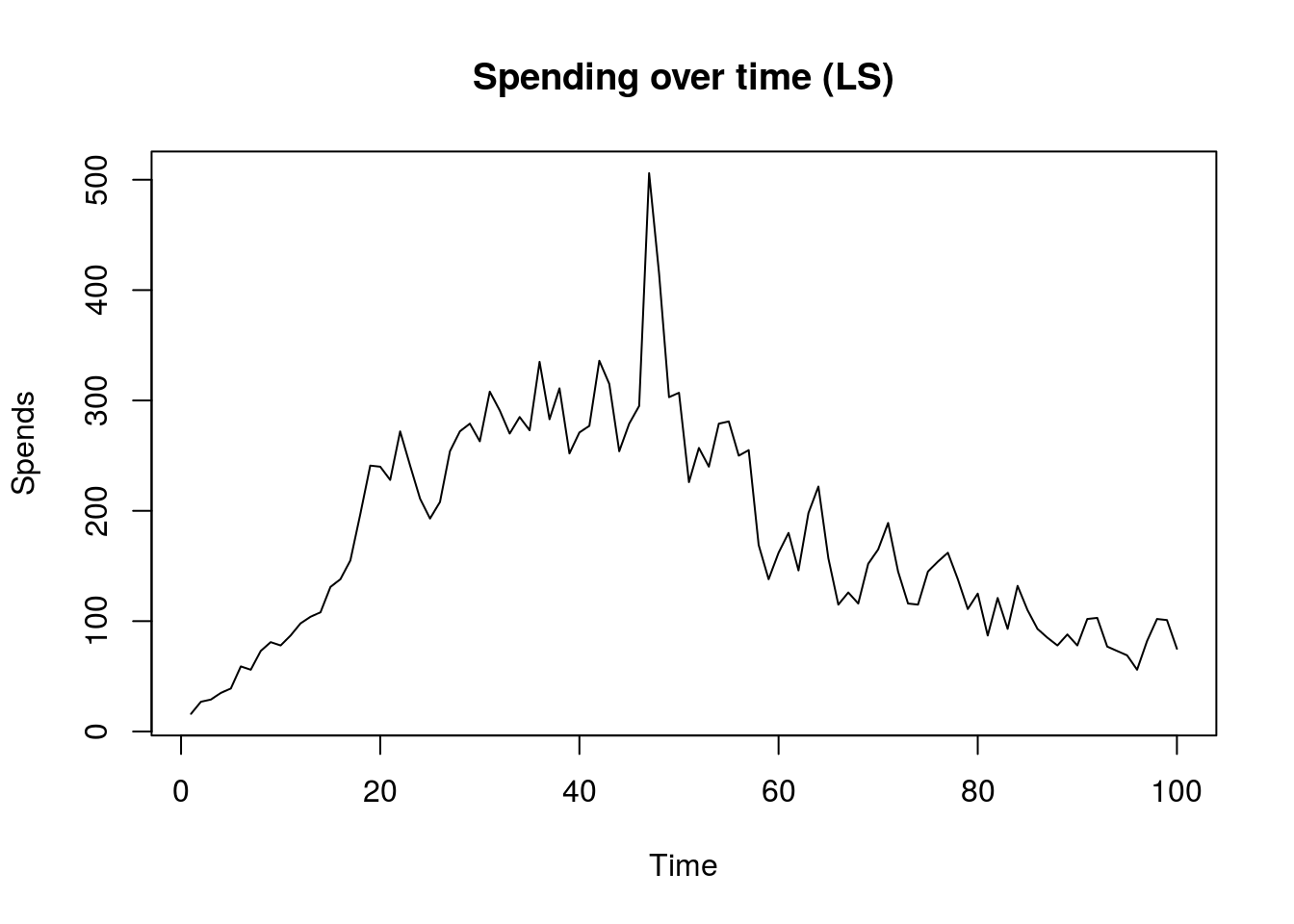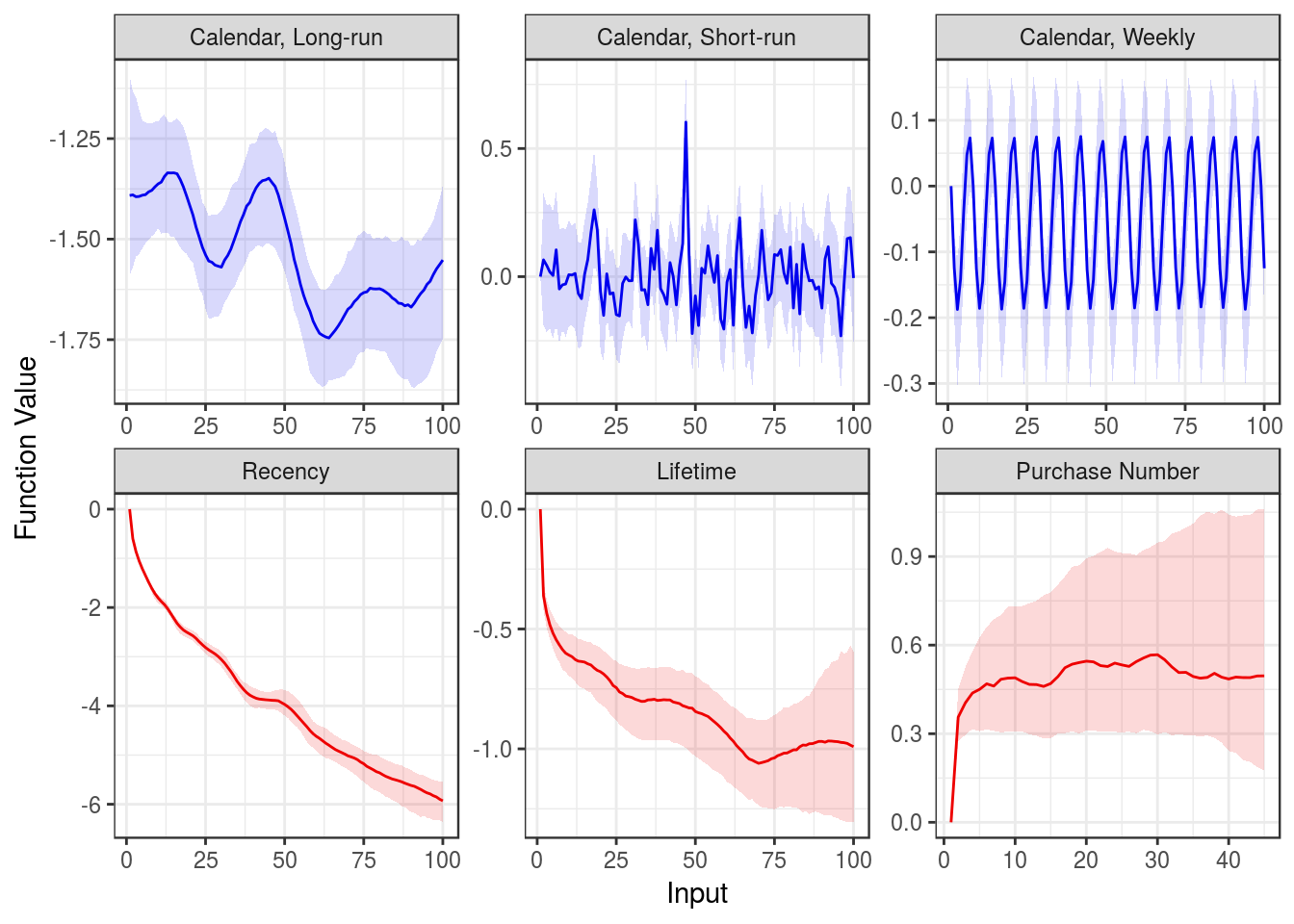This notebook accompanies the paper, Bayesian Nonparametric Customer Base Analysis with Model-based Visualizations, forthcoming at Marketing Science. In it, we illustrate the code for estimating the GPPM, and analyzing the results. We include the code needed to run the appendix simulations and the benchmark models separately in our replication files. Note that due to a non-disclosure agreement, we cannot use the true data for this illustration. Thus, we use simulated data that retains the general features of the true data.

We emphasize that all data used herein is synthetic, created by the authors via random number-based probabilistic simulation to exhibit similar aggregate patterns as in the data used in the paper.

## Prerequisites:

This notebook relies on several packages:

• `rstan` for estimation

• `foreach` and `doParallel` for multicore, embarassingly parallel simulations

• `mvtnorm` for simulating from the multivariate normal distribution

• `ggplot2` for plotting

``````require(rstan)
require(ggplot2)
require(mvtnorm)
require(doParallel)
require(foreach)``````

## Data summary:

The data take the form of a decomposed purchasing (spend incidence) event log, with columns indicating the calendar time day (`t`), the time since first spend (`l`, for lifetime), the time since most recent spend (`r`, for recency), the purchase number (`pnum`), and finally whether or not a spend actually occurred (`y`) at that combination of inputs, for person with a given ID (`id`). There is also a residual column indicating the day on which this person first spent (`fs`). For this illustration, we use the simulated data that is similar to the life simulator game:

``````data <- read.csv("ls_sim.csv")
``````##   y id fs  t r l pnum
## 1 0  1 20 21 1 1    2
## 2 0  1 20 22 2 2    2
## 3 0  1 20 23 3 3    2
## 4 0  1 20 24 4 4    2
## 5 0  1 20 25 5 5    2
## 6 0  1 20 26 6 6    2``````

From this, we can visualize the time series of spending, which we will fit later:

``````plot(as.vector(table(data[data[,"y"]==1,"t"])),
type="l", ylab="Spends", xlab="Time", main="Spending over time (LS)")``````## Estimation: Calling Stan

From the data, we create a list that can be used as input to Stan:

``````data_list <- list(M=nrow(data),
N=length(unique(data[,"id"])),
P=max(data[,"t"])-min(data[,"t"])+1,
K=max(data[,"pnum"])-min(data[,"pnum"])+1,
id=data[,"id"],
t=data[,"t"]-min(data[,"t"])+1,
l=data[,"l"],
r=data[,"r"],
pnum=data[,"pnum"]-min(data[,"pnum"])+1,
y=data[,"y"])``````

We normalize several of these to ensure they start at 1. The quantities in this list correspond directly to the quantities in the data block of the `gppm.stan` file, which is also included in the replication documents.

The code below calls the Stan sampler. NOTE: This may take a long time to run. We set the number of iterations here to be much smaller than those used in the paper, for illustration purposes. Note that the last two arguments to `stan()` just tell Stan to drop these intermediate quantities before saving the output. These variables are only used within the Stan program for normalization purposes:

``````fit <- stan(file="gppm.stan",
data=data_list,
warmup=200,  ## may need to be increased for proper convergence
iter=400,    ## may need to be increased for proper convergence
chains=1,
pars=c("free_week","free_short","free_rec",
"free_life","free_pnum","week_mean",
"short_mean","long_mean","rec_mean",
"life_mean","pnum_mean","z"),
include=FALSE,
seed=7926)

fit_list <- extract(fit)``````

Before sampling, there will appear several informational messages: these arise because of how we fix certain elements to zero for identification, as described in the paper, and can be ignored. We suppress them here in this notebook.

Once the Stan program finishes running, we extract the posterior samples with `extract()`, which we can use to create the model-based dashboards and tracking plots of interest. Because the Stan estimation can take a long time, we also include samples from a long run of the sampler, which can be loaded in lieu of running the model above. This loads the already computed `fit_list` object:

``````load("ls_fit.RData")

## Model-based Dashboard

The main output of interest of the GPPM is the model-based dashboard, which is simply a visualization of the latent function draws. It can be produced easily by plotting the posterior function draws:

``````dashdf <- with(fit_list, { rbind(data.frame(t=1:ncol(alpha_long),
plot="Calendar, Long-run",
med=apply(alpha_long,2,median),
lower=apply(alpha_long,2,quantile,probs=0.025),
upper=apply(alpha_long,2,quantile,probs=0.975)),
data.frame(t=1:ncol(alpha_short),
plot="Calendar, Short-run",
med=apply(alpha_short,2,median),
lower=apply(alpha_short,2,quantile,probs=0.025),
upper=apply(alpha_short,2,quantile,probs=0.975)),
data.frame(t=1:ncol(alpha_week),
plot="Calendar, Weekly",
med=apply(alpha_week,2,median),
lower=apply(alpha_week,2,quantile,probs=0.025),
upper=apply(alpha_week,2,quantile,probs=0.975)),
data.frame(t=1:ncol(alpha_rec),
plot="Recency",
med=apply(alpha_rec,2,median),
lower=apply(alpha_rec,2,quantile,probs=0.025),
upper=apply(alpha_rec,2,quantile,probs=0.975)),
data.frame(t=1:ncol(alpha_life),
med=apply(alpha_life,2,median),
lower=apply(alpha_life,2,quantile,probs=0.025),
upper=apply(alpha_life,2,quantile,probs=0.975)),
data.frame(t=1:ncol(alpha_pnum),
plot="Purchase Number",
med=apply(alpha_pnum,2,median),
lower=apply(alpha_pnum,2,quantile,probs=0.025),
upper=apply(alpha_pnum,2,quantile,probs=0.975))) })

colvec <- c(rep("blue2",3),rep("red2",3))
ggplot()+
geom_ribbon(data=dashdf,aes(x=t,ymin=lower,ymax=upper,fill=plot),alpha=0.15,color=NA)+
geom_line(data=dashdf,aes(x=t,y=med,color=plot))+
scale_color_manual(values=colvec)+
scale_fill_manual(values=colvec)+
facet_wrap(~plot,ncol=3,scales="free")+
ylab("Function Value")+
xlab("Input")+
theme_bw()+
theme(legend.position="none")``````We see the results in this simulated data are basically the same as in the paper, although there seems to be less separation between the short and long-run calendar time effects. Running the sampler for more iterations, or with slightly more informative priors, may alleviate that.

## Fit and Fit Decomposition

To see how the GPPM fits the data, we simulate spending according to the estimated propensities. To speed up this process, we parallelize these simulations:

``````cl <- makeCluster(detectCores()-1)
registerDoParallel(cl)

logit <- function(x){1/(1+exp(-x))}

N <- length(unique(data[,"id"]))
tmin <- min(data[,"t"])
P <- ncol(fit_list\$alpha_long)
niter <- nrow(fit_list\$alpha_long)

## This can be changed to only use a random subset of the total HMC iterations for simulation:
nrep <- nrow(fit_list\$alpha_long)

## Extract the posterior curves:
attach(fit_list)
iters <- sample(1:niter, nrep, replace=ifelse(nrep>niter,T,F))
alpha_long_rep <- alpha_long[iters,]
alpha_short_rep <- alpha_short[iters,]
alpha_week_rep <- alpha_week[iters,]
alpha_rec_rep <- alpha_rec[iters,]
alpha_life_rep <- alpha_life[iters,]
alpha_pnum_rep <- alpha_pnum[iters,]
delta <- delta[iters,]
detach(fit_list)

max_pnum <- ncol(alpha_pnum_rep)

rep_data <- foreach(i=1:N) %dopar% {
tmin_i <- min(data[data[,"id"]==i,"t"])-tmin+1
delta_i <- delta[,i]

irepdata <- array(NA,c(P,2,nrep))

ipt <- rep(1,nrep)
lt <- 1
pnum <- rep(1,nrep)

irepdata[1:(tmin_i-1),1,] <- 1:(tmin_i-1)
irepdata[1:(tmin_i-1),2,] <- 0

for(t in tmin_i:P){
ipind <- cbind(1:length(ipt),ipt)
pnumind <- cbind(1:length(pnum),ifelse(pnum>max_pnum,max_pnum,pnum))
irepdata[t,,] <- rbind(rep(t,nrep),rbinom(nrep,1,logit(alpha_long_rep[,t]+alpha_short_rep[,t]+
alpha_week_rep[,t]+
alpha_rec_rep[ipind]+
alpha_life_rep[,lt]+
alpha_pnum_rep[pnumind]+
delta_i)))

ipt <- ipt*(1-irepdata[t,2,])+1
pnum <- pnum+irepdata[t,2,]
lt <- lt+1
}

irepdata
}

## These just compile the simulation results:
combined <- do.call(rbind,lapply(rep_data, function(r){apply(r,3,function(x){x[,1]*x[,2]})}))
sim_sbd <- apply(combined, 2, function(x){table(factor(x[x>0], levels=1:P))})

# This computes the true spends-by-day in the data:
true_sbd <- as.vector(by(data[,"y"], data[,"t"], sum))

# This combines all of these to a data.frame that can be used by ggplot:
fitdf <- data.frame(t=rep(1:length(true_sbd),2),
points=c(true_sbd, apply(sim_sbd,1,median)),
curve=as.factor(c(rep(1,length(true_sbd)), rep(2,length(true_sbd)))),
lower=c(true_sbd, apply(sim_sbd,1,quantile,0.025)),
upper=c(true_sbd, apply(sim_sbd,1,quantile,0.975)))``````

Because these simulations can also take a while, especially without parallel processing, we again include an intermediate output file that contains the `fitdf` object:

``````# load("ls_fitplot_fitdf.RData")
``````ggplot(fitdf,aes(x=t,y=points,color=curve,linetype=curve))+